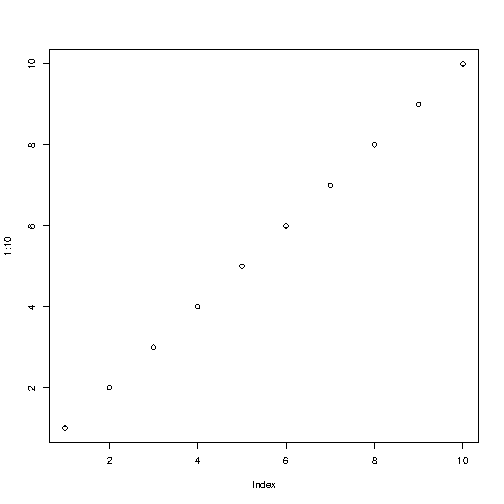# R Tutorial

R is a programming language.

R is often used for statistical computing and graphical presentation to analyze and visualize data.

Start learning R now »

## Examples in Each Chapter

With our "Try it Yourself" editor, you can edit R code and view the result.

### Example

How to output some text, and how to do a simple calculation in R:

"Hello World!"
5 + 5

Result:

```  "Hello World!"  10```
Try it Yourself »

### Example

How you can use R to easily create a graph with numbers from 1 to 10 on both the x and y axis:

plot(1:10)

Result:Try it Yourself »

We recommend reading this tutorial, in the sequence listed in the left menu.

## Exercise:

Insert the missing part of the code below to output "Hello World".

```Hello World
```

Start the Exercise

## R Examples

Learn by examples! This tutorial supplements all explanations with clarifying examples.

## R Quiz

Learn by taking a quiz! This quiz will give you a signal of how much you know about R.